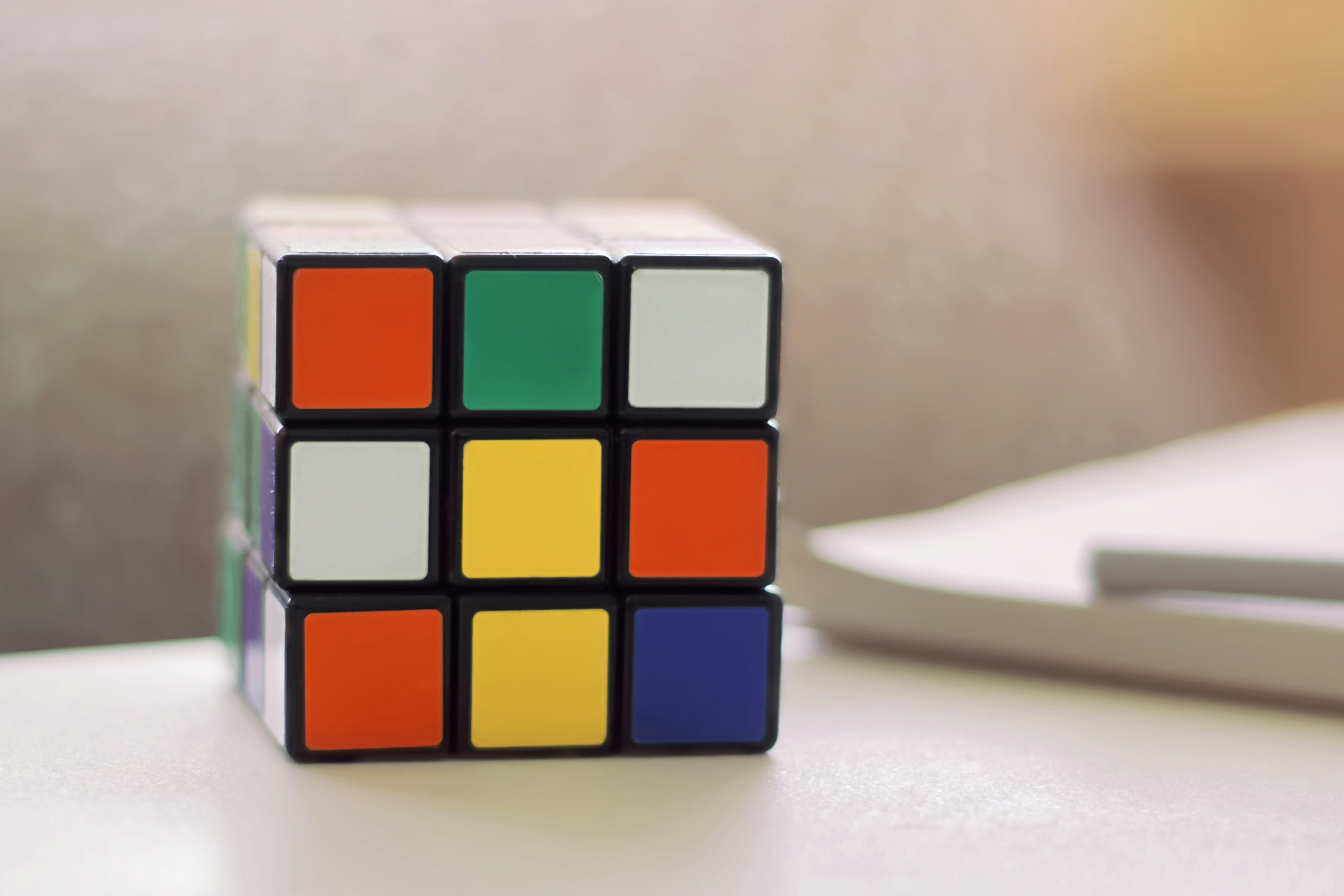## Before you go, check out these stories!

0What Exactly is Permutation and How to Calculate It? by@james-johnson

# What Exactly is Permutation and How to Calculate It?### @james-johnsonJames Johnson

I am a researcher and a technical content writer. A math teacher since 2007. I love travelling, Love

Ever heard of permutations? You wouldn’t be here if you weren’t interested, right? Well permutations are the number of configurations you can get out of a given set of values that require the factoring in of order.

So how exactly do you calculate them? Well, there are a number of ways you can use to achieve this purpose. However, first, let us break down the basics.

Okay, so the formula for the calculation of permutation goes like this:

nPr = n!/(n-r)!

Where,

P stands for permutation

n stands for the total number of values in a given set

r stands for the population extracted from the set

And,

! is the factorial

The ‘r’ part might confuse you if you are new to the concept. To make it easier for you to understand, consider a combination lock that requires three digits in a particular order to be unlocked. Now we all know that the entire foundation of math stands on 10 total digits from 0-9 that is.

This ‘0-9’ contains the total number of values in the set for the case of combination lock. In other words, this is our ‘n’. On the other hand, our lock requires three digits out of this set, in a particular order for it to be unlocked. This is our ‘r’.

So how many permutations would there be for a combination lock that unlocks with three digits out of a set of 10, in a particular order. Well, let’s put the formula to some use.

nPr = n!/(n-r)!

= 10!/(10-3)!

= 10!/7!

Putting the factorial in effect,

= 10*9*8*7*6*5*4*3*2*1/7*6*5*4*3*2*1

= 3628800/5040

= 720

There we have it. There are 720 possible permutations, out of which, the correct one, unlocks our combination lock, the probability of which would be 0.13 percent in case we forgot what the combination was. Safe to say, combination locks are quite secure by this estimation.

This is one method of figuring out the number of permutations. However, if you don’t want to waste your time going through all the math, you can always have fun with calculators like this one to get your answer.

Applications:

The above example might have cleared up on what kind of applications use permutations, the simpler ones are combination and digital locks, smart-phone pins and computer passwords. Permutations are heavily used in statistical mechanics, a branch of thermodynamics.

Permutations are also used for hashing in cryptography and in inter-leaver modules of error correcting algorithms like turbo code.

Permutation and combination: Difference

Reading the article, you might have confused permutations with combinations. That’s no surprise, since they’re essentially very similar, except for one major difference. ORDER! That’s right, combinations technically don’t care about the order. Combinations are also calculated differently.

For instance, there is no difference between 2,3,1, 1,2,3 and 3,1,2 when it comes to combinations. However, there is a difference between the above three sets of digits when it comes to permutation, due to order, which is the difference between all three sets.

Mathematically, the combination’s difference and similarity with the permutation is represented like this in the combination formula:

nCr = n!/r! (n-r)!

The formula is nearly the same as that for the permutation except that ‘C’ replaces ‘P’ and it stands for combination and r! accounts for the lack of order.Join Hacker Noon